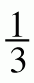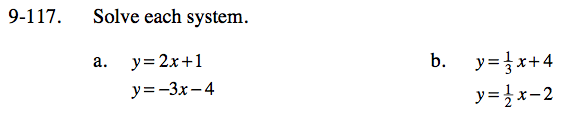Home > CC3 > Chapter 9 > Lesson 9.2.4 > Problem9-117

9-117.
1. Solve each system. Homework Help ✎

1. y = 2x + 1
y = −3x − 4

2. y =x + 4
y =x − 2Set the two equations equal to each other, then solve for x.

−3x − 4= 2x +1

−4 − 1= 2x + 3x

Use the method that you used to solve part (a).
See the hints for part (a) for more help.

(36, 16)